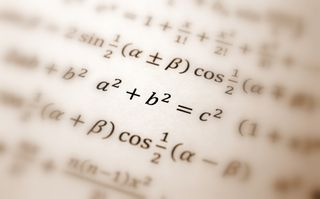# Mathematician Claims Proof of Connection between Prime NumbersA Japanese mathematician claims to have the proof for the ABC conjecture, a statement about the relationship between prime numbers that has been called the most important unsolved problem in number theory.

If Shinichi Mochizuki's 500-page proof stands up to scrutiny, mathematicians say it will represent one of the most astounding achievements of mathematics of the twenty-first century. The proof will also have ramifications all over mathematics, and even in the real-world field of data encryption.

The ABC conjecture, proposed independently by the mathematicians David Masser and Joseph Oesterle in 1985 but not proven by them, involves the concept of square-free numbers, or numbers that cannot be divided by the square of any number. (A square number is the product of some integer with itself). According to the mathematics writer Ivars Peterson in an article for the Mathematical Association of America, the square-free part of a number n, denoted by sqp(n), is the largest square-free number that can be obtained by multiplying the distinct prime factors of n. Prime numbers are numbers that can only be evenly divided by 1 and themselves, such as 5 and 17.

The ABC conjecture makes a statement about pairs of numbers that have no prime factors in common, Peterson explained. If A and B are two such numbers and C is their sum, the ABC conjecture holds that the square-free part of the product A x B x C, denoted by sqp(ABC), divided by C is always greater than 0. Meanwhile, sqp(ABC) raised to any power greater than 1 and divided by C is always greater than 1. [What Makes Pi So Special?]

This conjecture may seem esoteric, but for mathematicians, it's deep and ubiquitous. "The ABC conjecture is amazingly simple compared to the deep questions in number theory," Andrew Granville a mathematician at the University of Montreal, was quoted as saying in the MAA article. (Granville worked at the University of Georgia at the time.) "This strange conjecture turns out to be equivalent to all the main problems. It's at the center of everything that's been going on."

The conjecture has also been described as a sort of grand unified theory of whole numbers, in that the proofs of many other important theorems follow immediately from it. For example, Fermat's famous Last Theorem (which states that an+bn=cn has no integer solutions if n>2) follows as a direct consequence of the ABC conjecture.

In a 1996 article in The Sciences, the mathematician Dorian Goldfeld of Columbia University said the ABC conjecture "is more than utilitarian; to mathematicians it is also a thing of beauty. Seeing so many Diophantine problems unexpectedly encapsulated into a single equation drives home the feeling that all the subdisciplines of mathematics are aspects of a single underlying unity.

"No wonder mathematicians are striving so hard to prove it – like rock climbers at the base of a sheer cliff, exploring line after line of minute cracks in the rock face in the hope that one of them will offer just enough purchase for the climbers to pick their way to the top."

And now, one such climber may have reached the summit. According to Nature News, Mochizuki, a mathematician at Kyoto University, has proved extremely deep theorems in the past, lending credence to his claim that he has the proof for ABC. However, a huge investment of time by many other mathematicians will be required to go through the gargantuan proof and verify the claim.

"If the ABC conjecture yields, mathematicians will find themselves staring into a cornucopia of solutions to long-standing problems," Goldfeld wrote.

Editor's note: This article was corrected 9/14 at 11:50 a.m. to note that Andrew Granville now works at the University of Montreal. He worked at the University of Georgia at the time he made the statement quoted in the article.

This story was provided by Life's Little Mysteries, a sister site to LiveScience. Follow Natalie Wolchover on Twitter @nattyover or Life's Little Mysteries @llmysteries. We're also on Facebook & Google+.